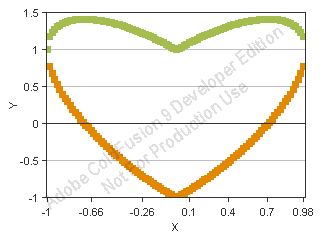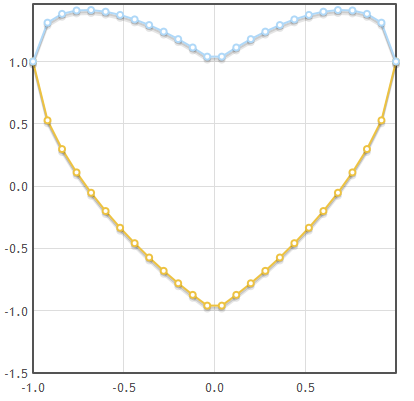## The formula of love plotted using ColdFusion and jQuery

Written on 13 March 2011, 11:55pm

Tagged with: , , , , ,

Did you know the formula of love? It’s
x^2+(y-sqrt(x^2))^2=1
Plotting this will result in a nicely hearth-shaped figure. The ultimate geek gift for his geek girlfriend!
I reproduced this plot using two methods: ColdFusion cfchart/cfchartseries/cfchartdata tags and a jQuery plotting library, called flot.

## 1. ColdFusion code

cflove.cfm (tested both on ColdFusion 8 and ColdFusion 9)

``````<cfchart format="flash" xaxistitle="X" yaxistitle="Y">
<cfchartseries type="scatter">
<cfloop index="x" from="-1" to="1" step="0.02">
<cfset y1 = sqr(x^2)-sqr(1-x^2)>
<cfchartdata item="#x#" value="#y1#">
</cfloop>
</cfchartseries>
<cfchartseries type="scatter">
<cfloop index="x" from="-1" to="1" step="0.02">
<cfset y2 = sqr(x^2)+sqr(1-x^2)>
<cfchartdata item="#x#" value="#y2#">
</cfloop>
</cfchartseries>
</cfchart>
``````

You will notice that the formula of love is composed by two curves:
-the upper part of the hearth: y = sqrt(x^2) – sqrt(1-x^2)
-the lower part of the hearth: y = sqrt(x^2) + sqrt(1-x^2)
The values of x are between -1 and 1.

Go ahead and load this file on your ColdFusion server. It will nicely draw the following image:## 2. jQuery code

flot.cfm (tested both on ColdFusion 8 and ColdFusion 9)

``````<html>
<style>
#chart {width:400px; height:400px;}
</style>
<script src="http://people.iola.dk/olau/flot/jquery.js"></script>
<script src="http://people.iola.dk/olau/flot/jquery.flot.js"></script>
<script>
\$.getJSON("flot.cfc?method=getplotdata&returnformat=json", {}, function(data) {
\$.plot(\$("#chart"),
[
{data:data},
{data:data}
]
,
{
lines:{show:true},
points:{show:true}
})
})
})
</script>

<body>
<div id="chart"></div>
</body>
</html>
``````

flot.cfc:

``````<cfcomponent>
<cffunction name="getPlotData" returnType="array" access="remote">

<cfset var result1 = []>
<cfset var result2 = []>
<cfloop index="x" from="-1" to="1" step="0.08">
<cfset x1 = x>
<cfset y1 = sqr(x^2)-sqr(1-x^2)>
<cfset x2 = x>
<cfset y2 = sqr(x^2)+sqr(1-x^2)>

<cfset point1 = [x1,y1]>
<cfset arrayAppend(result1, point1)>
<cfset point2 = [x2,y2]>
<cfset arrayAppend(result2, point2)>
</cfloop>

<cfset result = [result1,result2]>
<cfreturn result>
</cffunction>
</cfcomponent>
``````

The JavaScript files are loaded from the flot project examples homepage.
Pay attention to your ‘Prefix serialized JSON with’ setting in CF Admin. If it’s not checked, you should be fine. If you are using a prefix for your JSON data, make sure you strip it before passing it to getJSON function.
The result is this: